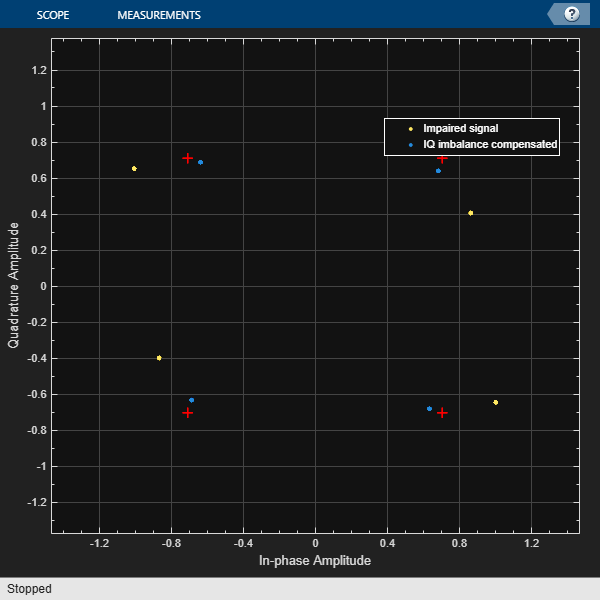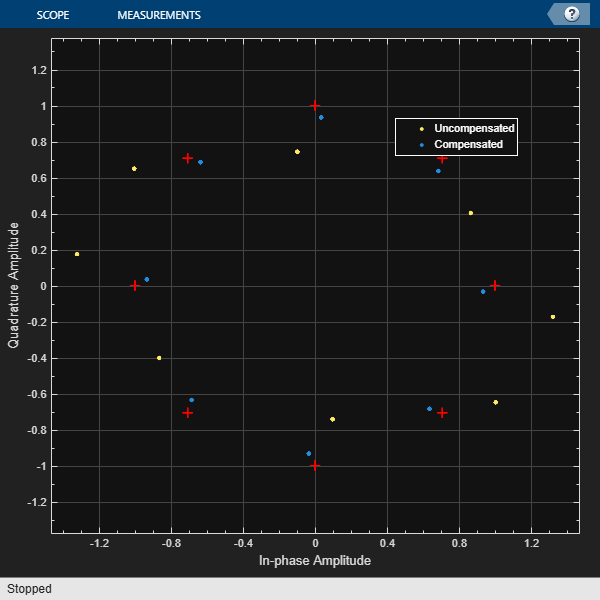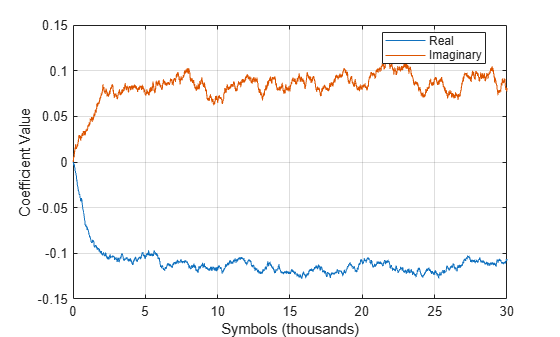# comm.IQImbalanceCompensator

Compensate for IQ imbalance

## Description

The `comm.IQImbalanceCompensator` System object™ compensates for the imbalance between the in-phase and quadrature (IQ) components of a modulated signal. The adaptive algorithm inherent to the IQ imbalance compensator is compatible with M-PSK, M-QAM, and OFDM modulation schemes, where M>2. For more information, see Algorithms.

Note

The output of the compensator might be scaled and rotated, that is, multiplied by a complex number, relative to the reference constellation. In practice, this transformation is not an issue because, before demodulation, receivers correct for it by using channel estimation.

To compensate for IQ imbalance:

1. Create the `comm.IQImbalanceCompensator` object and set its properties.

2. Call the object with arguments, as if it were a function.

## Creation

### Syntax

``iqcomp = comm.IQImbalanceCompensator``
``iqcomp = comm.IQImbalanceCompensator(Name=Value)``

### Description

example

````iqcomp = comm.IQImbalanceCompensator` creates a compensator System object that compensates for the imbalance between the in-phase and quadrature components of the input signal.```

example

````iqcomp = comm.IQImbalanceCompensator(Name=Value)` creates an IQ imbalance compensator object and sets properties using one or more name-value arguments. For example, `comm.IQImbalanceCompensator(CoefficientSource="Input port")` specifies that the compensator coefficients must be provided when you call the object. For this configuration, all other properties are disabled.```

## Properties

expand all

Unless otherwise indicated, properties are nontunable, which means you cannot change their values after calling the object. Objects lock when you call them, and the `release` function unlocks them.

If a property is tunable, you can change its value at any time.

Source of the compensator coefficients, specified as either ```"Estimated from input signal"``` or `"Input port"`.

• If this property is set to `"Estimated from input signal"`, the compensator uses an adaptive algorithm and the input signal to estimate the compensator coefficient.

• If this property is set to `"Input port"`, all other properties are disabled and the compensator coefficients must be provided as input argument `c` when you call the object.

Initial coefficient used to compensate for IQ imbalance, specified as a complex scalar.

#### Dependencies

To enable this property, set the `CoefficientSource` property to `"Estimated from input signal"`.

Data Types: `double`
Complex Number Support: Yes

Source of the step size to use for coefficient adaptation, specified as either `"Property"` or `"Input port"`.

• If this property is set to `"Property"`, specify the step size through the `StepSize` property.

• If this property is set to `"Input port"`, specify the step size as input argument `s` when calling the object.

Adaptation step size, specified as a scalar. The value of this property is the step size that the algorithm uses when estimating the IQ imbalance. For more information, see Algorithms.

Tunable: Yes

#### Dependencies

To enable this property, set the `StepSizeSource` to `"Property"`.

Data Types: `double`

Option to adapt the compensator coefficient from an input argument, specified as a logical `0` (`false`) or `1` (`true`).

• If this property is `false`, the coefficients update after each output sample.

• If this property is `true`, you must provide a logical value for the input argument `a` to enable and disable coefficient adaptation.

Option to output the compensator coefficients, specified as a logical `0` (`false`) or `1` (`true`).

• When this logical property is `false`, the IQ imbalance compensator coefficients are not available as output when you call the object.

• When this logical property is `true`, the IQ imbalance compensator coefficients are available as output `coef` when you call the object.

## Usage

### Syntax

``y = iqcomp(x)``
``y = iqcomp(x,c)``
``y = iqcomp(x,s)``
``y = iqcomp(x,a)``
``y = iqcomp(x,s,a)``
``[y,estcoef] = iqcomp(x,___)``

### Description

````y = iqcomp(x)` compensates for IQ imbalance of the input signal. The compensator coefficient is estimated from `x` by a blind adaptive algorithm.```
````y = iqcomp(x,c)` accepts input compensation coefficients, `c`, instead of generating them internally. This syntax applies when the `CoefficientSource` property is set to `Input port`. When using this syntax, adaptive estimation of the compensator coefficient is disabled.```
````y = iqcomp(x,s)` accepts input step size, `s`. This syntax applies when the `StepSizeSource` property is set to `Input port`.```
````y = iqcomp(x,a)` accepts the adaptation control signal, `a`, to enable or disable coefficient updates. This syntax applies when the `AdaptInputPort` property is set to `true`.```
````y = iqcomp(x,s,a)` accepts the step size, `s`, and the adaptation control signal, `a`, to enable and disable coefficient updates. This syntax applies when the `StepSizeSource` property is set to `Input port` and the `AdaptInputPort` property is set to `true`.```
````[y,estcoef] = iqcomp(x,___)` also returns estimated compensation coefficients with input arguments from any of the previous syntaxes. This syntax applies when the `CoefficientOutputPort` property is set to `Input port`.```

### Input Arguments

expand all

Input signal, specified as a scalar or a column vector.

Data Types: `double` | `single`
Complex Number Support: Yes

Compensator coefficients, specified as a scalar or a column vector the same length as input signal `x`.

#### Dependencies

To enable this input, set the `CoefficientSource` property to `"Input port"`.

Data Types: `single` | `double`

Step size for blind adaptive estimation of compensator coefficient, specified as a scalar.

#### Dependencies

To enable this input, set the `StepSizeSource` property to `"Input port"`.

Data Types: `single` | `double`

Adaptation control signal, specified as a logical scalar.

• If this property is `true`, then the compensator coefficient will be adapted.

• If this property is `false`, then the compensator coefficient will remain unchanged.

#### Dependencies

To enable this input, set the `AdaptInputPort` property to `"Input port"`.

Data Types: `logical`

### Output Arguments

expand all

IQ imbalance compensated signal, returned as a scalar or column vector with the same size as input signal `x`.

Estimated compensator coefficient, returned as a scalar or column vector with the same size as input signal `x`.

• If `a` is `true`, the returned compensator coefficient adapts when you call the object.

• If `a` is `false`, then the returned compensator coefficient remains unchanged from the last time you called the object.

## Object Functions

To use an object function, specify the System object as the first input argument. For example, to release system resources of a System object named `obj`, use this syntax:

`release(obj)`

expand all

 `step` Run System object algorithm `release` Release resources and allow changes to System object property values and input characteristics `reset` Reset internal states of System object

## Examples

collapse all

Mitigate the impacts of amplitude and phase imbalance on a QPSK modulated signal by using the `comm.IQImbalanceCompensator` System object™.

Generate random data symbols and apply QPSK modulation.

```M = 4; % QPSK spf = 1e6; % Samples per frame data = randi([0 M-1],spf,1); txSig = pskmod(data,M,pi/4);```

Create a constellation diagram object to display the QPSK signal before and after IQ imbalance compensation. The reference constellation for the object does not require an update because the default QPSK reference constellation matches the transmitted signal.

```cdscope = comm.ConstellationDiagram( ... NumInputPorts=2, ... ShowLegend=true, ... ChannelNames=["Impaired signal","IQ imbalance compensated"]);```

Create an IQ imbalance compensator.

`iqImbComp = comm.IQImbalanceCompensator;`

Apply amplitude and phase imbalance to the transmitted signal.

```ampImb = 5; % dB phImb = 15; % deg rxSig = iqimbal(txSig,ampImb,phImb);```

On the receiver side, apply the IQ compensation algorithm to the impaired signal.

`compSig = iqImbComp(rxSig);`

To display the impaired signal and the IQ impairment compensated signal, load the last 1000 symbols of the signals into the constellation diagram object. The impaired signal constellation shows IQ amplitude and phase impairments. The impairment compensated signal constellation nearly aligns with the reference constellation.

```cdscope(rxSig(spf - 1000:end),compSig(spf - 1000:end)) release(cdscope)```Compensate for an amplitude and phase imbalance on an 8-PSK signal by using the `comm.IQImbalanceCompensator` System object™ with external coefficients.

Generate an 8-PSK reference constellation. Create a constellation diagram System object. Configure the constellation diagram object to display only the last 100 data symbols of two input signals and provide the reference constellation.

```refconst = pskmod(0:7,8,0); cdscope = comm.ConstellationDiagram(... NumInputPorts=2, ... SymbolsToDisplaySource="Property", ... SymbolsToDisplay=100, ... ReferenceConstellation=refconst, ... ChannelNames=["Uncompensated","Compensated"]);```

Create an I/Q imbalance compensator object with an input port for the algorithm coefficients.

```iqcomp = comm.IQImbalanceCompensator( ... CoefficientSource="Input port");```

Generate random data symbols and apply 8-PSK modulation.

```data = randi([0 7],1000,1); txSig = pskmod(data,8,0);```

Apply amplitude and phase imbalance to the transmitted signal.

```ampImb = 5; % dB phImb = 15; % degrees rxSig = iqimbal(txSig,ampImb,phImb);```

Use the `iqimbal2coef` function to determine the compensation coefficient given the amplitude and phase imbalance.

`compCoef = iqimbal2coef(ampImb,phImb);`

Apply the compensation coefficient to the received signal when calling the `iqcomp` object. Display the resulting constellations for the uncompensated and compensated signal. You can see severe IQ imbalance in the uncompensated signal and the compensated signal constellation is nearly aligned with the reference constellation.

```compSig = iqcomp(rxSig,compCoef); cdscope(rxSig,compSig) release(cdscope)```Remove an IQ imbalance from a 64-QAM signal and make the estimated coefficients externally available while setting the algorithm step size from an input port.

Create a constellation diagram object. Use name-value pairs to ensure that the constellation diagram displays only the last 256 data symbols, set the axes limits, and specify the reference constellation.

```M = 64; refC = qammod(0:M-1,M); cdscope = comm.ConstellationDiagram(... NumInputPorts=2, ... SymbolsToDisplaySource="Property", ... SymbolsToDisplay=256, ... XLimits=[-10 10], ... YLimits=[-10 10], ... ReferenceConstellation=refC, ... ChannelNames=["Uncompensated","Compensated"]);```

Create an IQ imbalance compensator System object™ in which the step size is specified as an input argument and the estimated coefficients are made available through an output port.

```iqImbComp = comm.IQImbalanceCompensator( ... StepSizeSource="Input port", ... CoefficientOutputPort=true);```

Generate random data symbols and apply 64-QAM modulation.

```nSym = 30000; data = randi([0 M-1],nSym,1); txSig = qammod(data,M);```

Apply amplitude and phase imbalance to the transmitted signal.

```ampImb = 2; % dB phImb = 10; % deg rxSig = iqimbal(txSig,ampImb,phImb);```

Specify the step size parameter for the IQ imbalance compensator.

`stepSize = 1e-5;`

Compensate for the IQ imbalance while setting the step size with an input argument. Plot the constellation diagram of the received signal. You can see that the compensated signal constellation is now nearly aligned with the reference constellation.

```[compSig,estCoef] = iqImbComp(rxSig,stepSize); cdscope(rxSig,compSig) release(cdscope)```Plot the real and imaginary values of the estimated coefficients. The output coefficients show the simulation reaches a steady-state solution after approximately 5000 symbols.

```plot((1:nSym)'/1000,[real(estCoef),imag(estCoef)]) grid xlabel('Symbols (thousands)') ylabel('Coefficient Value') legend('Real','Imaginary','location','best')```Control the adaptation algorithm of the IQ imbalance compensator using an external argument.

Apply QPSK modulation to random data symbols.

```data = randi([0 3],600,1); txSig = pskmod(data,4,pi/4,'gray');```

Create an IQ imbalance compensator in which the adaptation algorithm is controlled through an input port, the step size is specified through the `StepSize` property, and the estimated coefficients are made available through an output port.

```iqImbComp = comm.IQImbalanceCompensator( ... AdaptInputPort=true, ... StepSize=0.001, ... CoefficientOutputPort=true);```

Apply amplitude and phase imbalance to the transmitted signal.

```ampImb = 5; % dB phImb = 15; % deg gainI = 10.^(0.5*ampImb/20); gainQ = 10.^(-0.5*ampImb/20); imbI = real(txSig)*gainI*exp(-0.5i*phImb*pi/180); imbQ = imag(txSig)*gainQ*exp(1i*(pi/2 + 0.5*phImb*pi/180)); rxSig = imbI + imbQ;```

Break the compensation operation into three segments in which the compensator is enabled for the first 200 symbols, disabled for the next 200 symbols, and enabled for the last 200 symbols. Save the coefficient data in three vectors.

```[~,estCoef1] = iqImbComp(rxSig(1:200),true); [~,estCoef2] = iqImbComp(rxSig(201:400),false); [~,estCoef3] = iqImbComp(rxSig(401:600),true);```

Concatenate the complex algorithm coefficients and plot their real and imaginary parts. Observe that the coefficients do not adapt when the compensator is disabled.

```estCoef = [estCoef1; estCoef2; estCoef3]; plot((1:600)',[real(estCoef) imag(estCoef)]) grid xlabel('Symbols') ylabel('Coefficient Value') legend('Real','Imaginary','location','best')```## Algorithms

Imbalance between the in-phase and quadrature components of signal output from RF receivers can be cost-effectively compensated rather than improving the analog front-end RF hardware. Direct conversion receivers, in particular, introduce IQ imbalance. A circularity-based blind compensation algorithm is used as the basis for the IQ imbalance compensator.

A generalized IQ imbalance model is shown, where g is the amplitude imbalance and ϕ is the phase imbalance. For no impairment, g = 1 and ϕ = 0. In the figure, H(f) is the nominal frequency response of the branches due to, for example, low-pass filters. HI(f) and HQ(f) represent the portions of the in-phase and quadrature amplitude and phase responses that differ from the nominal response. With perfect matching, HI(f) = HQ(f) = 1.Let z(t) be the ideal baseband equivalent signal of the received signal, r(t), where its Fourier transform is denoted as Z(f). Given the generalized IQ imbalance model, the Fourier transform of the imbalanced signal, x(t) = xI(t) + xQ(t), is

`$X\left(f\right)={G}_{1}\left(f\right)Z\left(f\right)+{G}_{2}\left(f\right){Z}^{*}\left(-f\right)$`

where G1(f) and G2(f) are the direct and conjugate components of the IQ imbalance. These components are defined as

`$\begin{array}{l}{G}_{1}\left(f\right)=\left[{H}_{I}\left(f\right)+{H}_{Q}\left(f\right)g\mathrm{exp}\left(-j\varphi \right)\right]/2\\ {G}_{2}\left(f\right)=\left[{H}_{I}\left(f\right)+{H}_{Q}\left(f\right)g\mathrm{exp}\left(j\varphi \right)\right]/2\end{array}$`

Applying the inverse Fourier transform to X(f), the signal model becomes x(t) = g1(t) × z(t) + g2(t) × z*(t).

This transformation suggests the compensator structure as shown in which discrete-time notation expresses the variables. The compensated signal is expressed as y(n) = x(n) + wx*(n).An algorithm of the form

`$\left\{\begin{array}{l}y\left(n\right)=x\left(n\right)+w\left(n\right){x}^{*}\left(n\right)\\ w\left(n+1\right)=w\left(n\right)-M{y}^{2}\left(n\right)\end{array}$`

is used to determine the weights because it ensures that the output is proper, that is, the condition, E[y2(n)] = 0 is satisfied. For further details, see . The initial value of w is determined by the initial compensator coefficient, which has a default value of `0 + 0i`. M is the adaptation step size as described in `StepSize`.

 Anttila, L., M. Valkama, and M. Renfors. "Blind compensation of frequency-selective I/Q imbalances in quadrature radio receivers: Circularity-based approach", Proc. IEEE ICASSP, pp.III-245–248, 2007.

 Kiayani, A., L. Anttila, Y. Zou, and M. Valkama, "Advanced Receiver Design for Mitigating Multiple RF Impairments in OFDM Systems: Algorithms and RF Measurements", Journal of Electrical and Computer Engineering, Vol. 2012.